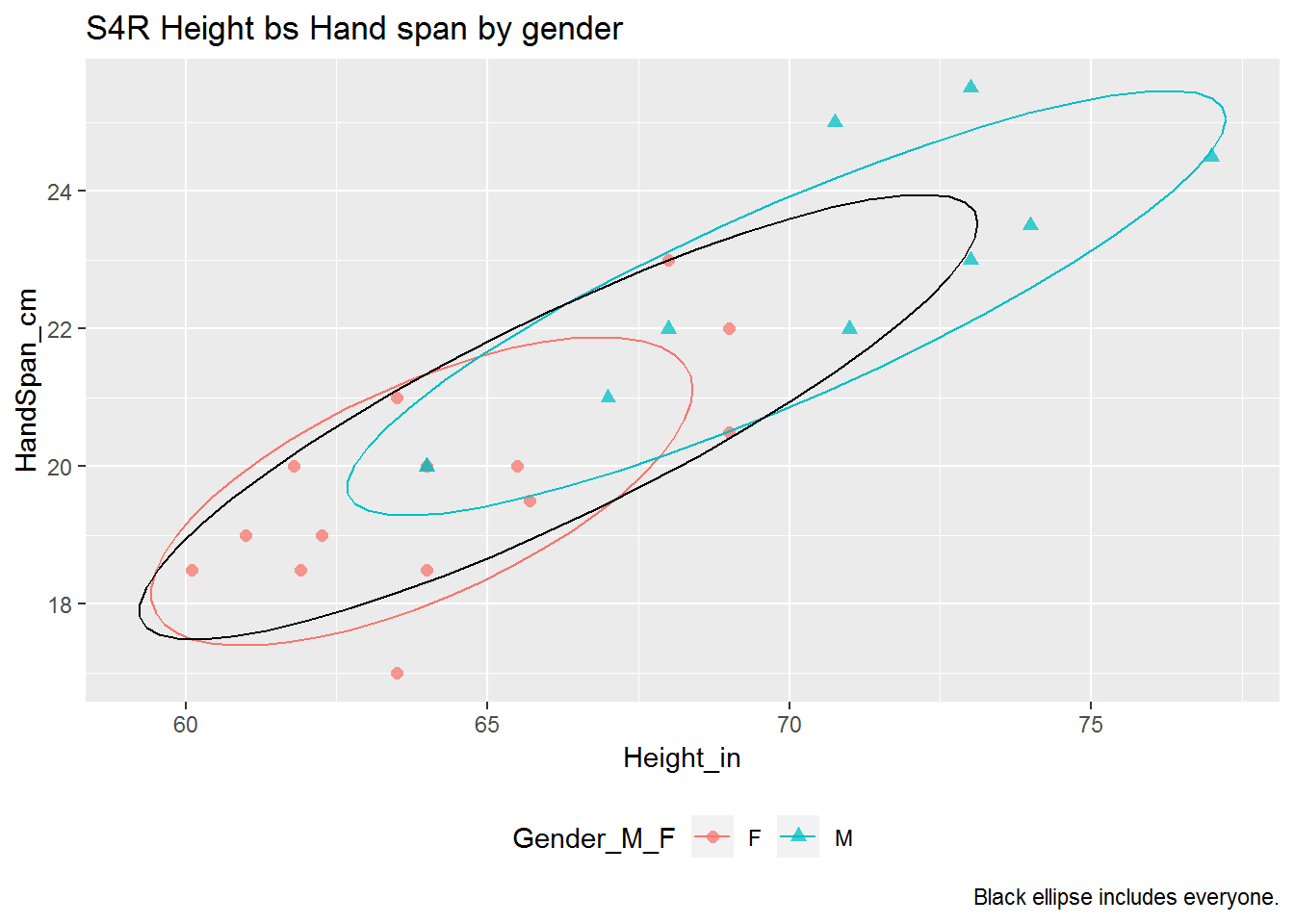This assignment is separate from your project. Include your answers in this document in the sections below the rubric.

Answer the questions with the correlation data example.

Rubric

Refer to the numerical summaries and plots below to answer the questions in the rubric up here.

1. (2 p) Participate in two data collection and entering activities. Describe any potential issues about the data collection process.

2. (3 p) Interpret correlation for Males, Females, and Everyone combined.

3. (2 p) How would the correlation change if both hand span and height were measured in inches?

4. (3 p) Why is there a difference in the strength of the correlation for everyone compared to either gender separately?

Height vs Hand Span

Procedure:

1. Record your height in inches. For example 5’0" is 60 inches.
2. Use a ruler to measure your hand span in centimeters: the distance from the tip of your thumb to pinky finger with your hand splayed as wide as possible.
3. Record your gender, height, and hand span on the whiteboard.
4. Erik will upload the data to the website.
5. Analysis and interpretation.
library(tidyverse)
## -- Attaching packages ------------- tidyverse 1.2.1 --
## v ggplot2 3.1.0       v purrr   0.3.2
## v tibble  2.1.1       v dplyr   0.8.0.1
## v tidyr   0.8.3       v stringr 1.4.0
## v readr   1.3.1       v forcats 0.4.0
## -- Conflicts ---------------- tidyverse_conflicts() --
## x dplyr::filter() masks stats::filter()
## x dplyr::lag()    masks stats::lag()
dat.hand <-
## Parsed with column specification:
## cols(
##   Table = col_double(),
##   Person = col_double(),
##   Gender_M_F = col_character(),
##   Height_in = col_double(),
##   HandSpan_cm = col_double()
## )
dat.hand <- na.omit(dat.hand)
dat.hand$Gender_M_F <- factor(dat.hand$Gender_M_F, levels = c("F", "M"))

str(dat.hand)
## Classes 'tbl_df', 'tbl' and 'data.frame':    24 obs. of  5 variables:
##  $Table : num 1 1 1 1 1 1 2 2 2 2 ... ##$ Person     : num  1 2 3 4 5 6 1 2 3 4 ...
##  $Gender_M_F : Factor w/ 2 levels "F","M": 1 2 1 1 1 1 1 1 2 1 ... ##$ Height_in  : num  64 73 61.9 63.5 60.1 61 69 69 71 65.5 ...
##  \$ HandSpan_cm: num  18.5 25.5 18.5 17 18.5 19 22 20.5 22 20 ...
##  - attr(*, "na.action")= 'omit' Named int  7 8 9 18 26 27 31 32 33 34 ...
##   ..- attr(*, "names")= chr  "7" "8" "9" "18" ...
# correlation by gender
dat.hand %>%
group_by(Gender_M_F) %>%
summarize(
corr = cor(Height_in, HandSpan_cm, use = "complete.obs")
)
## # A tibble: 2 x 2
##   Gender_M_F  corr
##   <fct>      <dbl>
## 1 F          0.675
## 2 M          0.840
# correlation for everyone
dat.hand %>%
summarize(
corr = cor(Height_in, HandSpan_cm)
)
## # A tibble: 1 x 1
##    corr
##   <dbl>
## 1 0.866
library(ggplot2)
p <- ggplot(dat.hand, aes(x = Height_in, y = HandSpan_cm, shape = Gender_M_F, colour = Gender_M_F, group = Gender_M_F))
p <- p + geom_point(alpha = 0.75, size = 2)
p <- p + stat_ellipse(type = "t", level = 0.75)
# ellipse for everyone by setting "group = 1"
p <- p + stat_ellipse(aes(group = 1), colour = "black", type = "t", level = 0.75)
p <- p + labs(
title = "S4R Height bs Hand span by gender"
, caption = "Black ellipse includes everyone."
)
p <- p + theme(legend.position = "bottom")
print(p)